# Volume of Prisms and Cylinders The volume of

• Slides: 18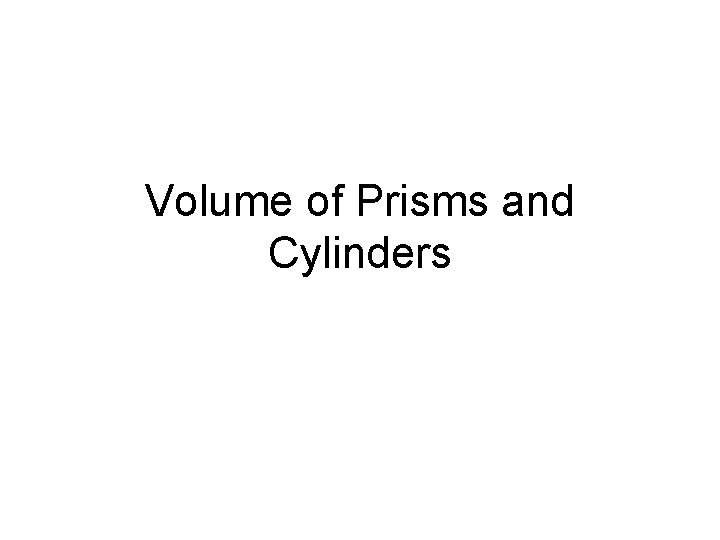Volume of Prisms and Cylinders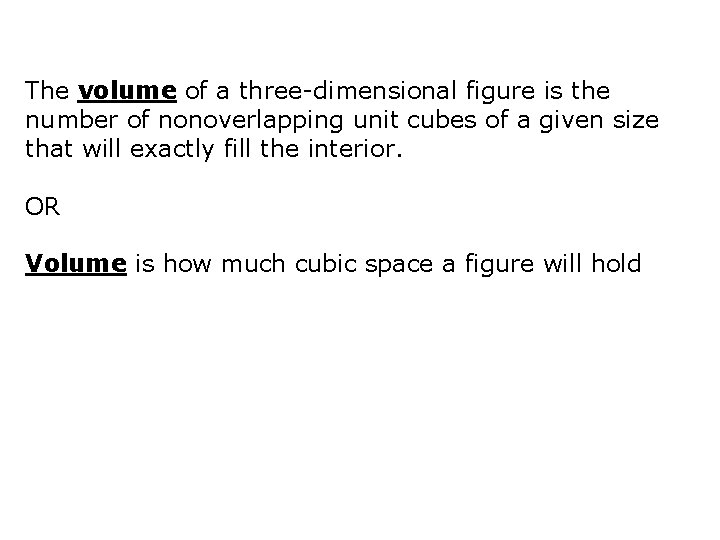The volume of a three-dimensional figure is the number of nonoverlapping unit cubes of a given size that will exactly fill the interior. OR Volume is how much cubic space a figure will hold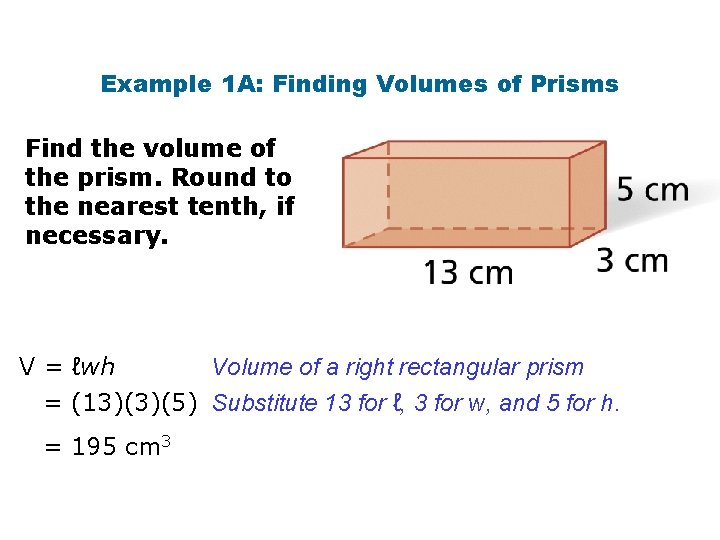Example 1 A: Finding Volumes of Prisms Find the volume of the prism. Round to the nearest tenth, if necessary. Volume of a right rectangular prism V = ℓwh = (13)(3)(5) Substitute 13 for ℓ, 3 for w, and 5 for h. = 195 cm 3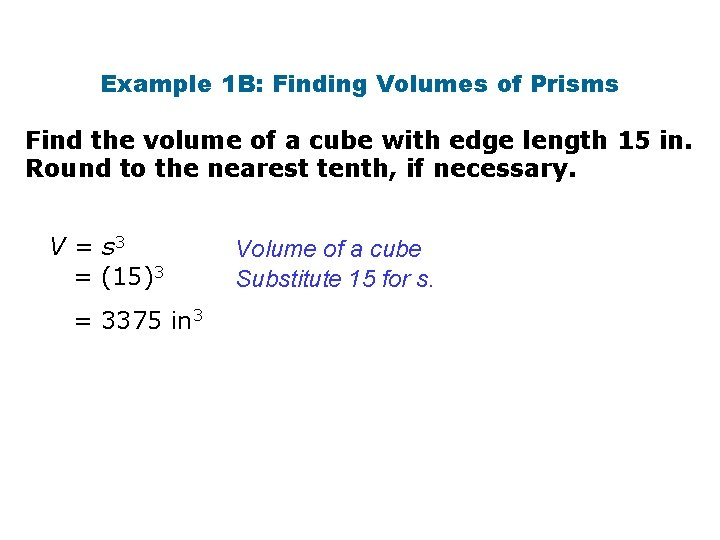Example 1 B: Finding Volumes of Prisms Find the volume of a cube with edge length 15 in. Round to the nearest tenth, if necessary. V = s 3 = (15)3 = 3375 in 3 Volume of a cube Substitute 15 for s.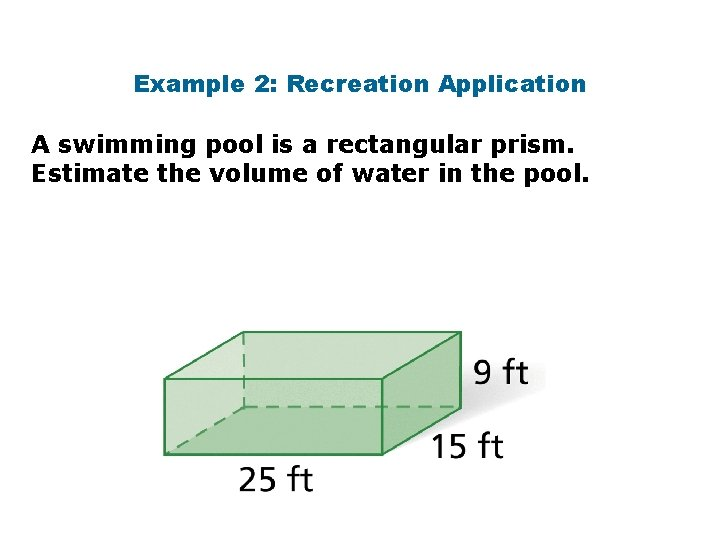Example 2: Recreation Application A swimming pool is a rectangular prism. Estimate the volume of water in the pool.Example 2 Continued Find the volume of the swimming pool in cubic feet. V = ℓwh = (25)(19) = 3375 ft 3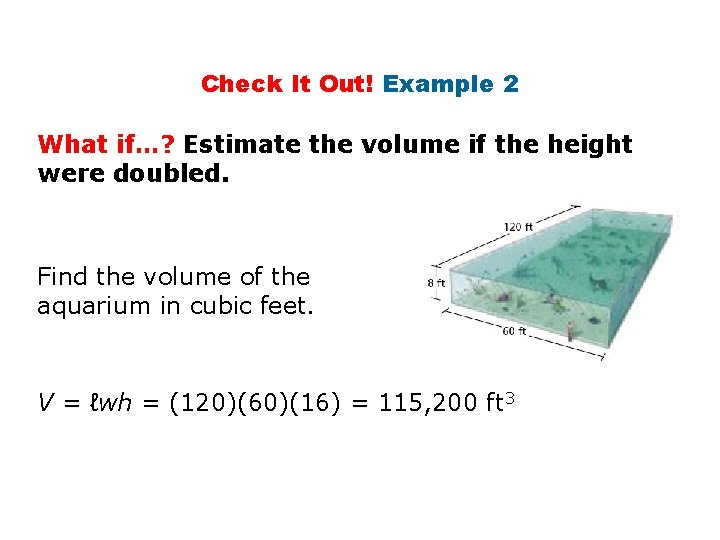Check It Out! Example 2 What if…? Estimate the volume if the height were doubled. Find the volume of the aquarium in cubic feet. V = ℓwh = (120)(60)(16) = 115, 200 ft 3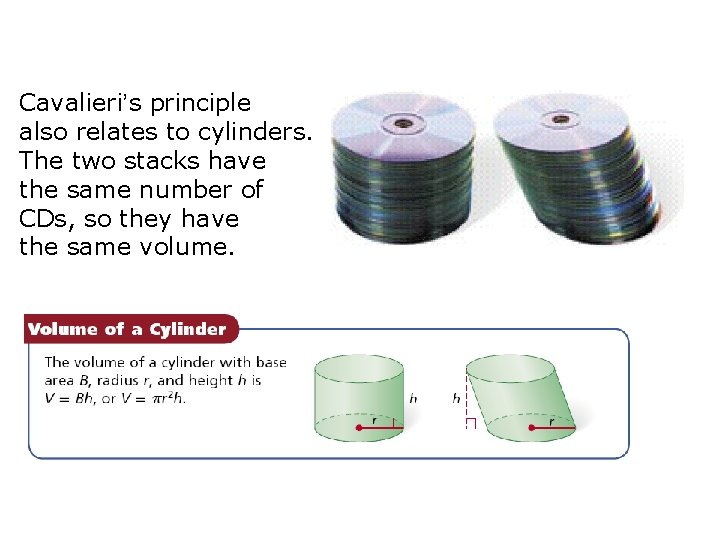Cavalieri’s principle also relates to cylinders. The two stacks have the same number of CDs, so they have the same volume.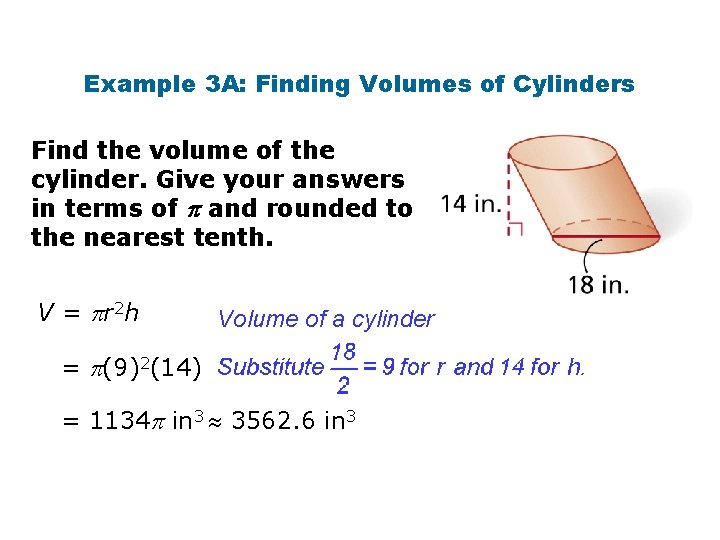Example 3 A: Finding Volumes of Cylinders Find the volume of the cylinder. Give your answers in terms of and rounded to the nearest tenth. V = r 2 h Volume of a cylinder = (9)2(14) = 1134 in 3 3562. 6 in 3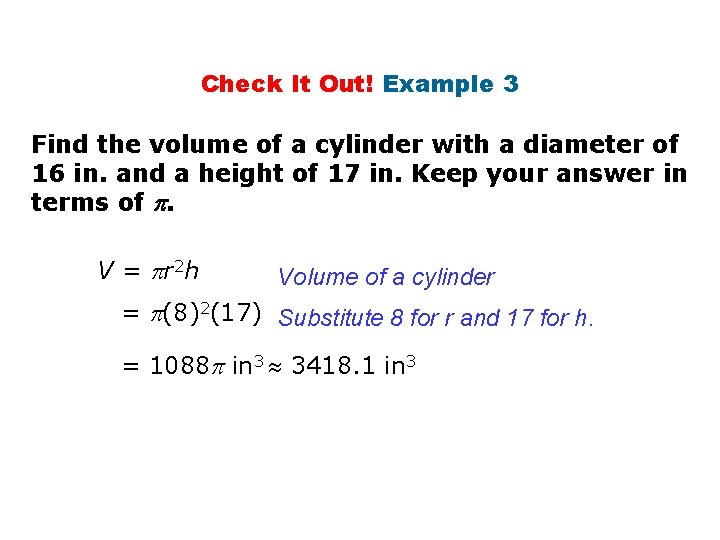Check It Out! Example 3 Find the volume of a cylinder with a diameter of 16 in. and a height of 17 in. Keep your answer in terms of . V = r 2 h Volume of a cylinder = (8)2(17) Substitute 8 for r and 17 for h. = 1088 in 3 3418. 1 in 3Example 4: Exploring Effects of Changing Dimensions The radius and height of the cylinder are multiplied by. Describe the effect on the volume. original dimensions: radius and height multiplied by :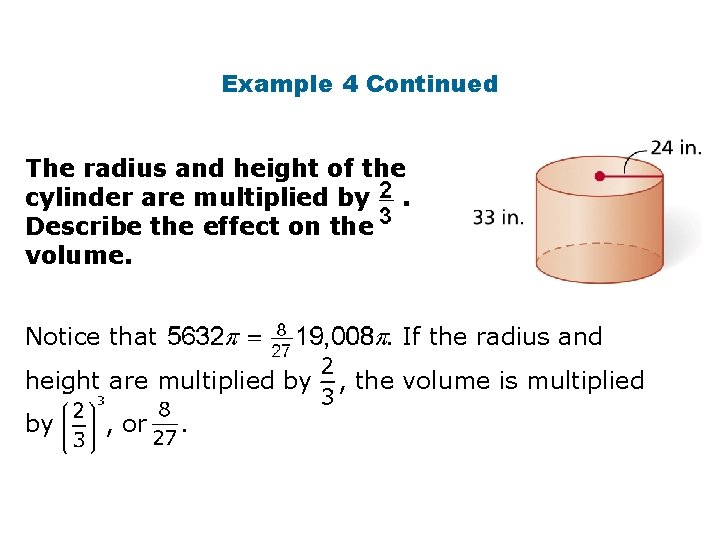Example 4 Continued The radius and height of the cylinder are multiplied by. Describe the effect on the volume. Notice that . If the radius and height are multiplied by by , or . , the volume is multiplied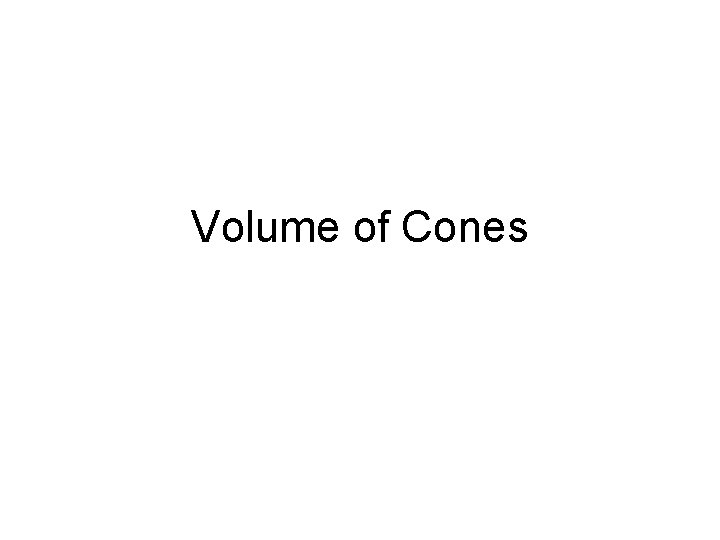Volume of Cones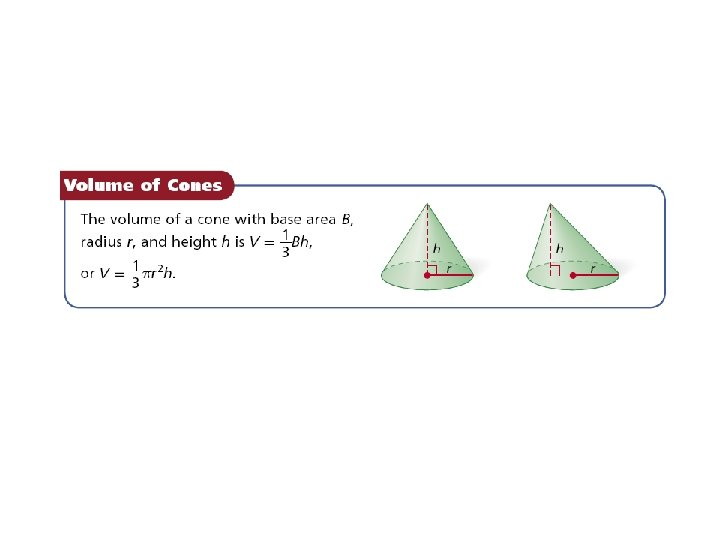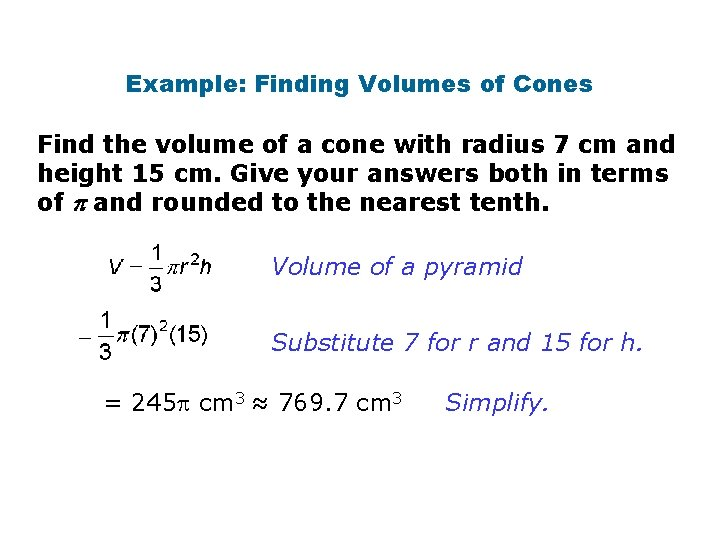Example: Finding Volumes of Cones Find the volume of a cone with radius 7 cm and height 15 cm. Give your answers both in terms of and rounded to the nearest tenth. Volume of a pyramid Substitute 7 for r and 15 for h. = 245 cm 3 ≈ 769. 7 cm 3 Simplify.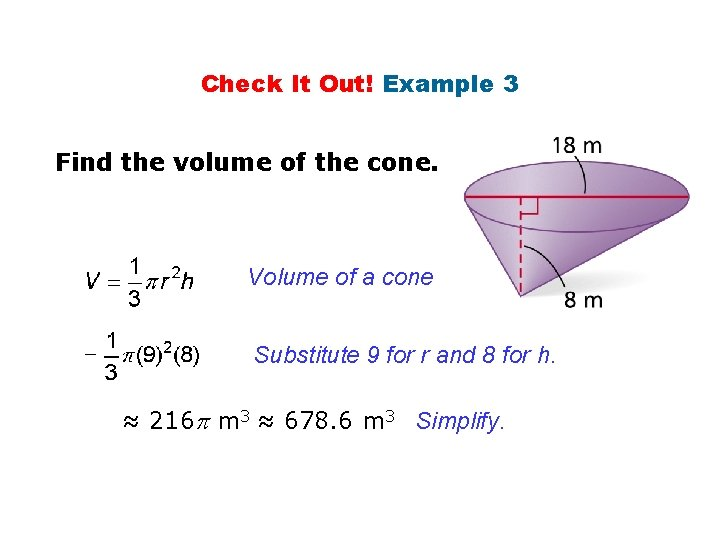Check It Out! Example 3 Find the volume of the cone. Volume of a cone Substitute 9 for r and 8 for h. ≈ 216 m 3 ≈ 678. 6 m 3 Simplify.Homework • • • Page 83, #3, 5 Page 85, #13 Page 86, #15, 17 Page 87, #20, 21, 26 Page 88, #29 Page 89, #37 a, 38 a